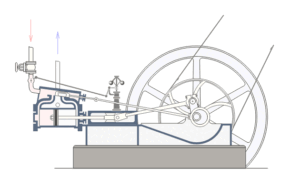# Classical Physics I – Energy, Power and Boiling Water

##The Concept of Energy

Energy is one of those broad words, which encapsulate a full variety of everyday meanings: “I haven’t got the energy to keep on walking.  My laptop battery has run out of energy.  The cost of energy is going up again…”

‘Energy’ is a concept with a precise scientific meaning, and one of the most important concepts in the whole of Science.  Energy is a physical property possessed by an object.  Energy is a measure of the capability of that object to ‘make things happen’.  And for ‘things to happen’, some of the energy of the object needs to be transferred to some other objects.

One type of energy is inherent to the motion of an object – kinetic energy.

Another type of energy comes from food.  It can be processed by the body and makes it possible for our muscles to function – chemical energy.

Energy also has an effect on temperature.  Burning gas under a saucepan of water transfers the chemical energy from the gas combustion into flame (light and heat) that results into an increase of temperature of the water – thermal energy or heat.

Indeed, a common consequence of transferring  energy to an object is to cause its temperature to rise.

## The Joule J, Ubiquitous Energy Unit

As energy is a physical property possessed by an object, it is possible to measure it.  Although energy exists in many different forms, it can all be measured using the same unit.

The SI unit of energy is called the joule, named after British scientist James Prescott Joule (1818-1889) who contributed in developing our understanding of energy.

The symbol for the joule is$J$.

The joule is a rather small unit of energy by everyday standards.  As an example, when an electric kettle is used to heat water, energy is transferred to the heating element from the current circulating through it.  This energy is then transferred from the heating element to the water.  An electric kettle holds about two litres of water.  If the kettle is well insulated, so as to avoid heat loss, and the water gets heated from$20^{\circ} C$ (ambient temperature) to$100^{\circ} C$, then about$6.7 \times 10^5 J$ of energy will have been transferred from the heating element of the kettle to the water.

## Heat Transfer and the Concept of Power

Just like energy, power is another of those “buzz” words with everyday meanings that has a precise scientific meaning.  This meaning is derived from the scientific concept of energy.

Power is in fact the rate at which an energy transfer takes place, i.e. the amount of energy transferred in one unit of time.

The word formula is simple:$power = energy \: transferred \: per \: unit \: of \: time$.

To put it another way,$power = \frac {energy \: transferred}{time}$.

Now, we have seen that the SI unit of energy is the joule,$J$, the SI unit of time is the second,$s$.Therefore, the SI unit of power is the Joule per second, that is$J s^{-1}$.  But because it is rather cumbersome to refer to the rate of energy transfer or power in terms of joules per second, an equivalent unit is often used in order to specify the power requirements of electrical appliances: the watt,$W$.

The watt is a general unit for the rate of all forms of energy transfer, named after Scottish engineer James Watt (1736-1819), a major contributor to the Industrial Revolution, who made significant improvements to the design of steam engines, the main source of technological power of his day.

## On the Dry Subject of Boiling Water

Suppose that 500 joules of energy are transferred from one object to another in 20 seconds.  The transfer of energy per second is then$\frac {500 J}{20 s} = 25 J / s$ or$25 J s^{-1}$.

This is the rate of energy transfer, or power.

In many circumstances, it is the rate of energy transfer that matters most, not the total amount that is transferred.

For example, if we want to know how  rapidly the temperature of a litre of water will rise by$1^{\circ} C$, it is not enough to know that 4200 J will elevate from$20^{\circ} C$ to$21^{\circ} C$.

We must find out how quickly the energy is transferred, or supplied, to the water.

Now, 4200 J is the amount of energy that is required to raise the temperature by$1^{\circ} C$.  If it is supplied every second, the temperature rise will also take one second.  But if it is supplied over two seconds, the temperature will take two seconds to rise by$1^{\circ} C$.  If the energy is supplied over 0.1 second, the temperature rise will only take 0.1 s.

For each of these cases,

• If 4200 J are supplied in 1.0 s, the rate of energy supply (power) is 4200 joules per second, i.e.$4200 J s^{-1}$.
• If 4200 J are supplied in 2.0 s, the rate of energy transfer is$\frac {4200 J}{2.0 s} = 2100 J s^{-1}$.  As expected, the power is halved since it takes twice as long to achieve the heat transfer.
• If 4200 J are supplied in 0.1 s, the rate of energy supply is$\frac {4200 J}{0.10 s} = 42,000 J s^{-1}$.  As expected, the rate of energy supply in this case is 10 times higher that in the first case.